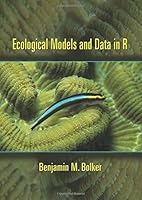# Ecological Models and Data in R## Book Description

Ecological Models and Data in R is the first truly practical introduction to modern statistical methods for ecology. In step-by-step detail, the book teaches ecology graduate students and researchers everything they need to know in order to use maximum likelihood, -theoretic, and Bayesian techniques to analyze their own data using the R. Drawing on extensive experience teaching these techniques to graduate students in ecology, Benjamin Bolker shows how to choose among and construct statistical models for data, estimate their parameters and confidence limits, and interpret the results. The book also covers statistical frameworks, the philosophy of statistical modeling, and critical mathematical functions and probability distributions. It requires no programming background--only basic calculus and statistics.

• Practical, beginner-friendly introduction to modern statistical techniques for ecology using the programming language R
• Step-by-step instructions for fitting models to messy, real-world data
• Balanced view of different statistical approaches
• Wide coverage of techniques--from simple ( fitting) to complex (state-space modeling)
• Techniques for data manipulation and display
• Companion Web site with data and R for all examples

Chapter 1. Introduction
Chapter 2. Exploratory data analysis and graphics
Chapter 3. Deterministic functions for ecological modeling
Chapter 4. Probability and stochastic distributions for ecological
Chapter 5. Stochastic simulation and power analysis
Chapter 6. Likelihood and all that
Chapter 7. Optimization and all that
Chapter 8. Likelihood examples
Chapter 9. Standard statistics revisited
Chapter 10. Modeling variance
Chapter 11. models
Chapter 12. Afterword
Appendix A. Algebra and calculus basics

## Book Details

• Title: Ecological Models and Data in R
• Author:
• Length: 408 pages
• Edition: 1
• Language: English
• Publisher:
• Publication Date: 2008-07-21
• ISBN-10: 0691125228
• ISBN-13: 9780691125220